Name:    Geometry Chapter 7 - Right Triangles and Trigonometry

1.

Find the length of the leg of this right triangle. Give an approximation to 3 decimal places.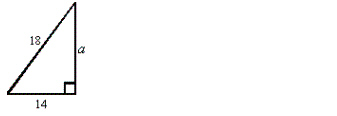a. 10.863 b. 22.804 c. 11.314 d. 5.657

2.

Which set of lengths cannot form a right triangle?
 a. 4.5 mm, 6 mm, 7.5 mm c. 10 mm, 12 mm, 15 mm b. 9 mm, 12 mm, 15 mm d. 18 mm, 24 mm, 30 mm

3.

A photographer shines a camera light at a particular painting forming an angle of 47° with the camera platform. If the light is 52 feet from the wall where the painting hangs, how high above the platform is the painting?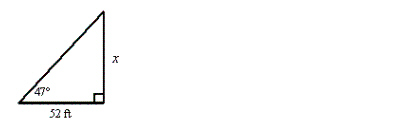a. 1.07 ft b. 48.49 ft c. 0.93 ft d. 55.76 ft

4.

Write cos A.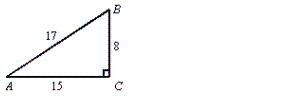a.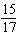b.c.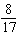d.5.

A slide 3.8 m long makes an angle of 25° with the ground. How high is the top of the slide above the ground?
 a. 1.77 m b. 1.61 m c. 1.66 m d. 3.44 m

6.

What is x to the nearest hundredth? (not drawn to scale)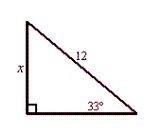a.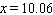b.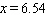c.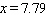d.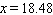7.

Solve for x to the nearest degree.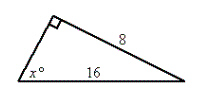a. 30 b. 60 c. 63 d. 27

8.

Find the length of the altitude drawn to the hypotenuse.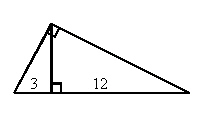a. 36 b. 9 c. 15 d. 6

9.

If the short leg of a 30-60-90 triangle is 4, what is the length of the hypotenuse?
 a.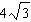b. 8 c. 4 d.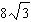10.

If the hypotenuse of a 45-45-90 triangle is 16, what is the length of one of its legs?
 a.b.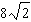c. 4 d. 8